# Area and Perimeter Worksheets

#### What is Area and Perimeter?

Mensuration is a part of geometry. It helps in solving shape-related problems and comes in very handy in everyday lives. The two most basic elements of mensuration are area and perimeter. Perimeter is the measure of the total length of the boundary of a 2D shape. The Sum of all sides is how you can calculate the perimeter of a shape. When talking about the circle, the measure of the total length of the boundary is what we term as the circumference. The area is the measure of the space enclosed within the boundary of a 2D shape. There are different formulae to calculate the area of different shapes.

Square: l2
Rectangle: L × B
Triangle: 1/2 × base × height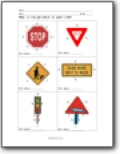What is the perimeter of each road sign item?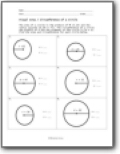###### Visual Area / Circumference of a Circle

The area of a circle is the product of π (3.14) and the radius squared (3.14 x r2). The circumference of a circle the product of π and the diameter of the circle (3.14 x D). Find the area and circumference for each circle below.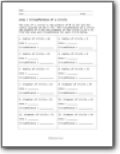###### Area / Circumference From Measures

You will be given a radius and asked to determine both of these measures for both circles.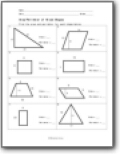###### Area/Perimeter of Mixed Shapes

Find the area and perimeter for each shape below.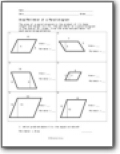###### Area/Perimeter of a Parallelogram

The area of a parallelogram is the product of its base length and height (b x h). The perimeter of a parallelogram is the sum of all sides. Find the area and perimeter for each parallelogram below.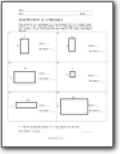###### Area/Perimeter of a Rectangle

The area of a rectangle is the product of its length and width (l x w). The perimeter of a rectangle is the sum of all sides. Find the area and perimeter for each rectangle below.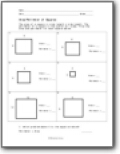###### Area/Perimeter of Squares

The area of a square is side length x side length. The perimeter of a square is the sum of all sides. Find the area and perimeter for each square below.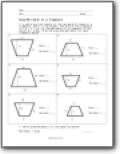###### Area/Perimeter of a Trapezoid

If a and b are the lengths of the two parallel bases of a trapezoid, and h is its height, the area of the trapezoid is 1/2 × h × (a + b).The perimeter of a trapezoid is the sum of all sides. Find the area and perimeter for each trapezoid below.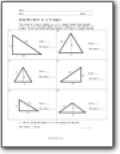###### Area/Perimeter of a Triangle

The area of the triangle is 1/2 × base length and height (1/2 x b x h).The perimeter of a triangle is the sum of all sides. Find the area and perimeter for each triangle below.